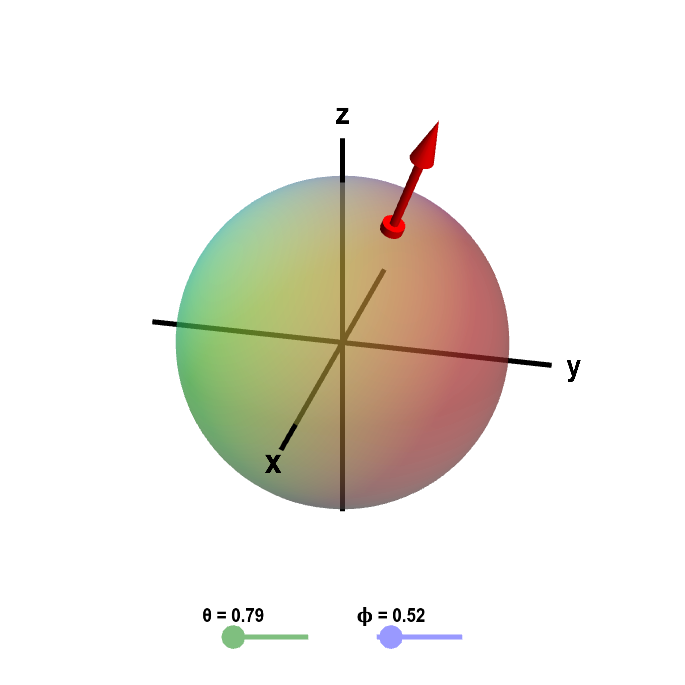# Math Insight

### Applet: Sphere with outward normal vectorThe sphere of a fixed radius $R$ is parametrized by $\dlsp(\theta,\phi) = (R\sin \phi \cos\theta, R\sin\phi \sin\theta, R\cos\phi)$ for $0 \le \theta \le 2\pi$ and $0 \le \phi \le \pi$. In this case, we have chosen the outward pointing normal vector $\vc{n} = (\sin \phi \cos\theta, \sin\phi \sin\theta, \cos\phi),$ orienting the surface so the outside is the positive side. You can change the values of $\theta$ and $\phi$ by moving the base of the normal vector or by dragging the points on the sliders.